Project

# Create a PID Controller on the NI myRIO—The Software

November 29, 2016 by Mark Narvidas

## In this short project article series, we will implement a simple PID controller using LabVIEW on a hobby DC servo motor and the NI myRIO.

Learn about the software side of this PID controller project using LabVIEW and an NI myRIO.

### Related Information

In this series, we discuss how to implement a simple PID controller using LabVIEW and a hobby DC servo motor. We customize the servo by removing its internal controller and replacing it with a myRIO interface.

This is the second article where we build upon our customized servo motor and focus on the programming of the PID controller exclusively. You will need to follow the first part of the series, which discusses the project's hardware, to implement the steps shown below.

### LabVIEW

LabVIEW is a graphical programming language developed by National Instruments. One of the greatest benefits of the language is the vast array of toolkits, modules, and libraries that are available for it. Using these, you can quickly develop most complex applications for a multitude of engineering and research projects.

This experiment is no exception. Instead of writing our PID controller completely from scratch, we will take full control of the NI LabVIEW PID and Fuzzy Logic Toolkit. Using the regular “PID.VI” that ships with the software package, we will be able to solve our challenge of bypassing the original servo controller much more quickly.

This tutorial is not intended to cover nitty-gritty theoretical details of PID. Instead, it will show you how to implement a simple PID controller.

### Requirements

To follow, you should have the following available and/or installed:

• NI myRIO embedded hardware device
• NI myRIO Expansion Port (MXP) Protoboard Accessory (comes pre-packed with myRIO)
• Customised servo motor which should by now be connected to the Protoboard Accessory
• NI LabVIEW
• NI LabVIEW PID and Fuzzy Logic Toolkit

In this tutorial, I use NI LabVIEW version 2014 to program the NI myRIO device with the NI LabVIEW PID and Fuzzy Logic Toolkit installed. If you are still a student, you should be able to acquire all the software and relevant licenses from your university.

### Servo Control—Theory vs. Practice

Previously, we explained how a servo is controlled and how we bypass the internal controller of the device to control velocity instead of the position. We said that these devices accept pulse-width modulation (PWM) signals (Figure 1) and follow the standard RC hobby servo protocol.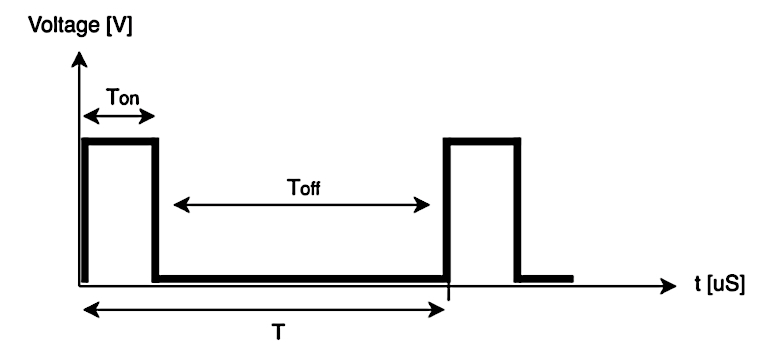##### Figure 1: Simple servo control through PWM

According to this standard protocol, a regular servo will move to its leftmost position at a pulse length (TON) of 1000 μs and rightmost at 2000 μs, staying in the middle at 1500 μs (Table 1).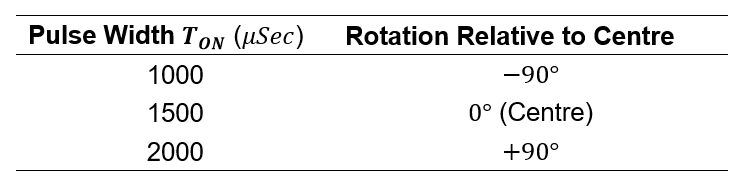##### Table 1: Standard servo behavior

When customized, it should then move fastest to the left at 1000 μs, to the right at 2000 μs, and remain stationary at 1500 μs (Table 2). Note that these assumptions assume a 50 Hz refresh rate.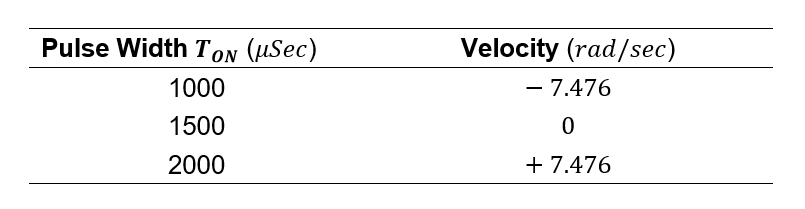##### Table 2: Servo behavior after customization

Nevertheless, in practice, these values can vary quite drastically, especially with budget servos like the one that we use in this tutorial.

Therefore, we will empirically determine the center position, ourselves, and then add arbitrary positive and negative offsets for clockwise and counterclockwise travel.

### Software

#### Creating a LabVIEW Project

First, let’s create a LabVIEW project. Fire up LabVIEW and, on the splash screen, select "Create Project" (alternatively, you can go to File >> Create Project).

On the new pop-up window, select “myRIO Project” and click “Next”.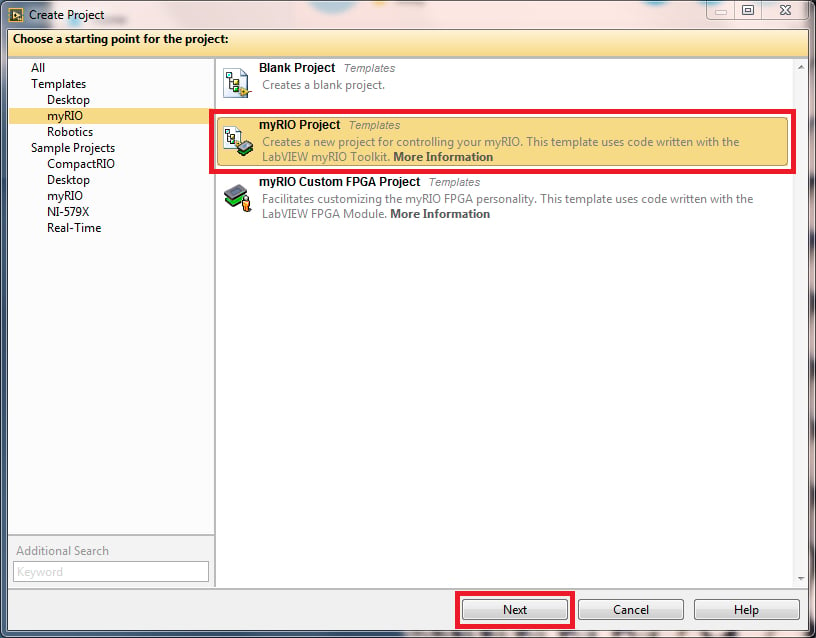##### Figure 2: Creating a myRIO project – step 1

Then give the project a name. I called mine simply “PID.”

Finally, make sure the myRIO is plugged in and found, at which point you can click “Finish.”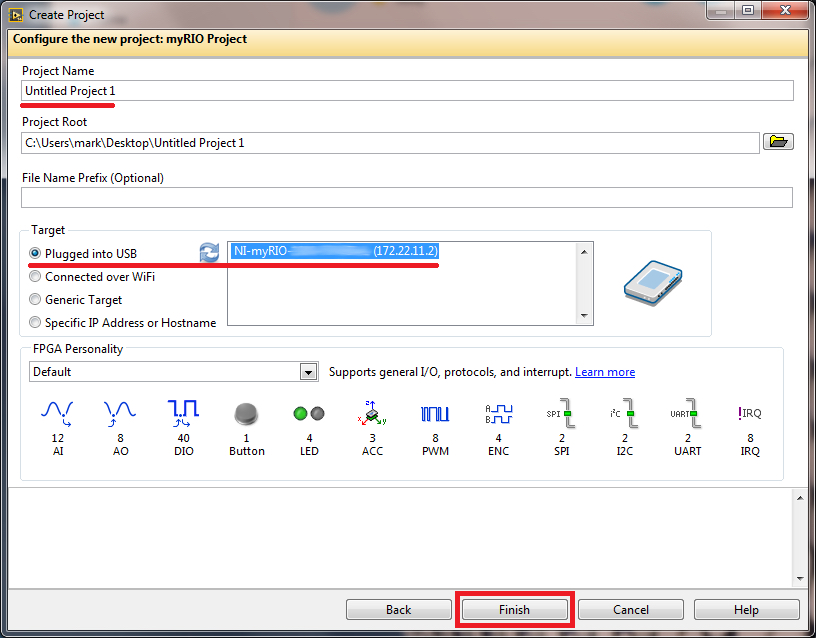#### Create a New VI

Then, create a new VI called “PID Controller.VI” under the myRIO target as shown in Figure 4. You can ignore or even delete the “Main.VI”—it gets created by default as an example for every myRIO project created under this template.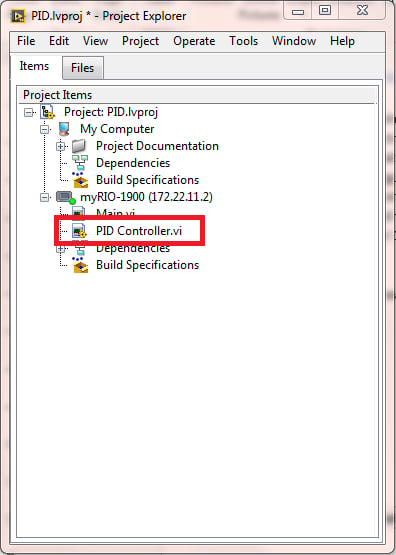##### Figure 4: Creating a new VI on the myRIO

Open the new VI and switch to the Block Diagram window. Create a while loop with an associated stop button. This is where we will put all of our code that needs to be executed continuously.##### Figure 5: While loop with a stop button

Next, using Quick Drop (CTRL+Space) or selecting the function from myRIO function palette, add “Analog Input” express VI as shown in Figure 6.

Within the configuration pop-up window, ensure that Channel selected is “A/AI0 (Pin 3)” and give the channel a name. I called it "Feedback" because, using this function block, we will read in our position values from the internal servo potentiometer.

Then click “OK” and place the express VI within the while loop.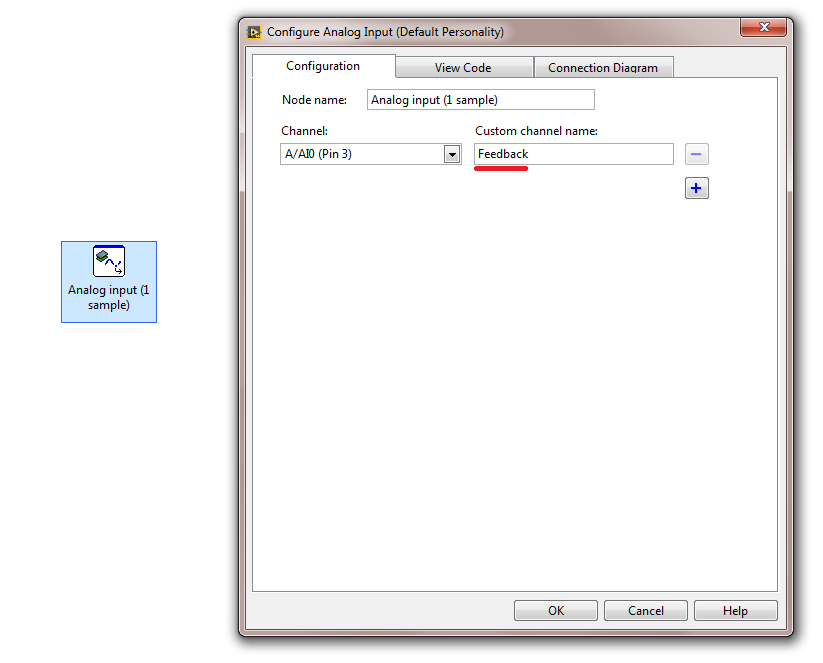Similarly, let’s create a PWM output so we can control the servo velocity. Again, either using Quick Drop or the function palette, add an express VI called “PWM” (Figure 7).

For your channel, select “A/PWM0 (Pin 27).” Set your frequency to 50 Hz (via the "Set constant" option) and select “Set using input to Express VI” under “Duty cycle.”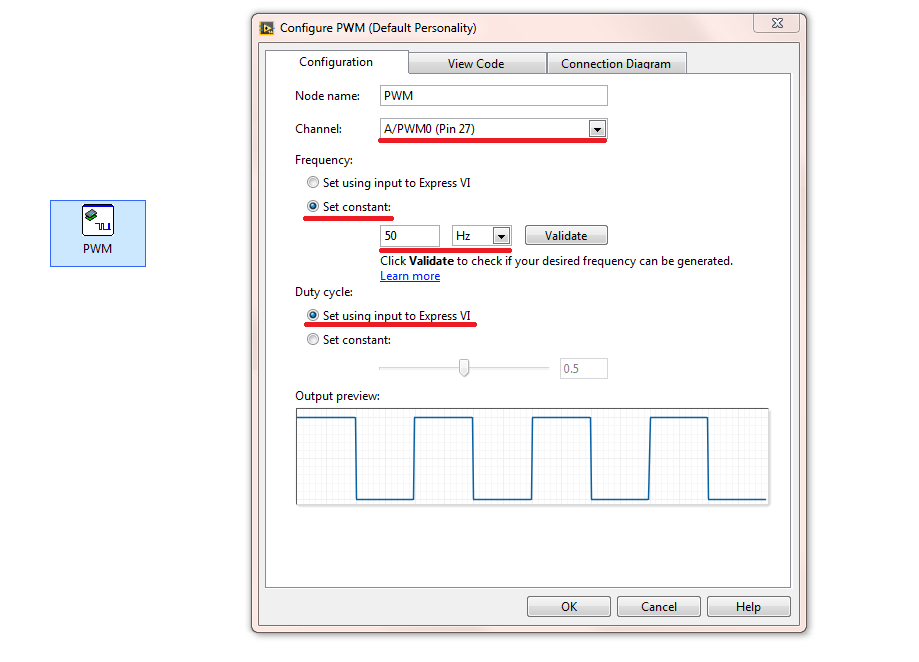##### Figure 7: Adding PWM functionality

Then click “OK” and put the block within a while loop. You should have a layout similar to the one shown in Figure 8.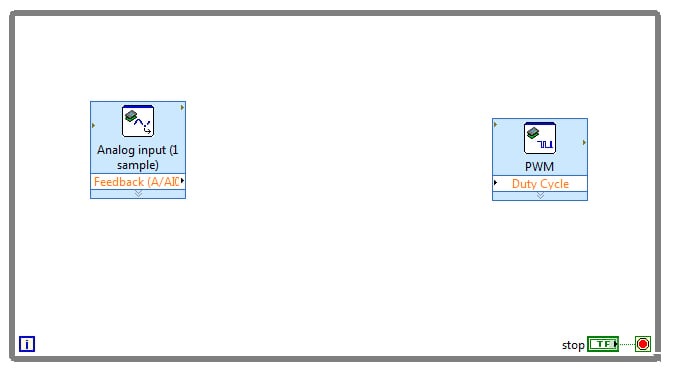#### Determining the Servo's Center Position

Next, we will aim to determine the center position of the servo. To do that, create a control for the “duty cycle” input.

You can create an indicator for "Feedback" as well so you can observe which value the potentiometer is at (it will range between 0 and 5, but as we will see later it is simple to convert these values into a different range). We will also add some timing with the “Wait” function. The block diagram is shown in Figure 9; the Wait function is displayed as a wristwatch, and the constant 10 attached to it means that we have 10 ms of delay.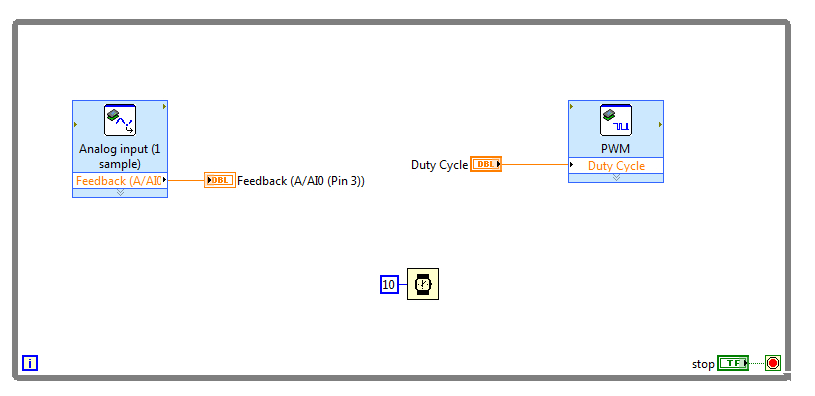##### Figure 9: Adding controls, indicators, and timing.

Theoretically, our duty cycle will range between pulse widths of 1 ms and 2 ms, with 1.5 ms being the value which stops the servo (zero velocity). Our 50 Hz signal has a period of 20 ms, so these pulse widths correspond to duty cycles of 0.05, 0.10, and 0.075.

In practice, the actual pulse width that produces zero velocity can vary from the theoretical value. To find the actual value, run the VI with the duty cycle set to 0.075 and then adjust it until the servo does not rotate. In my case, the actual value was 0.058 (Figure 10).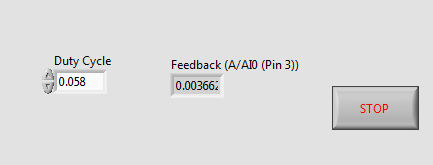##### Figure 10: Front panel of the center-finding code (Front Panel).

Next, we will add arbitrary velocity-control offsets to this center value.

I chose an offset of 0.012. This means that the maximum angular speed in the clockwise direction corresponds to a duty cycle of 0.046 (0.058 - 0.012), and similarly the maximum angular speed in the counterclockwise direction corresponds to 0.070 (0.058 + 0.012). These mappings are shown in Table 3.##### Table 3: Velocity mappings

Any intermediate values represent a fraction of the maximum angular speed in a given direction. For example, for counterclockwise rotation at half of the maximum speed, the controller would need to set the duty cycle to 0.058 + (0.012/2) = 0.064.

#### PID Controller Implementation

Once we determine the middle position, we can finally implement the PID controller. Using either Quick Drop or the function palette, add the “PID.vi” from the LabVIEW PID and Fuzzy Logic toolkit.

Create a control for the setpoint input; the setpoint is the value (in this system, a rotational velocity) that we want the output to have.

We can also create a control for the PID gains and the output range (leave these unchanged for now).

You should now have a VI similar to the one shown in Figure 11.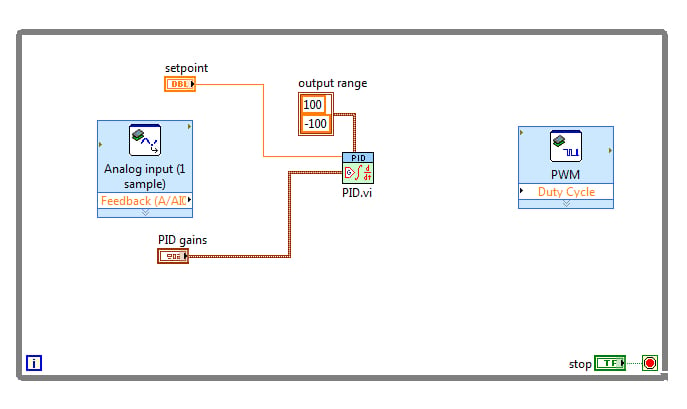##### Figure 11: Adding PID control.

We need to ensure that our setpoint, output, and feedback vary over an appropriate range of values.

Our output range is set to vary between -100 and 100 (Figure 11), so let’s apply this same range to the feedback. Feedback is initially in the range 0 to 5, and thus we need to scale these values (Table 4).##### Table 4: Feedback mapping table; 0 should map to -100, and 5 to 100.

Scaling is accomplished by applying a slope (denoted by A) and an offset (denoted by B) to the feedback values. We can find the required slope and offset by solving the following two simultaneous equations: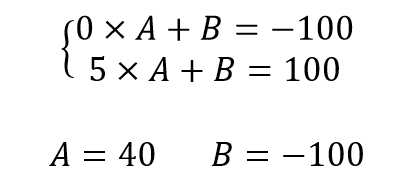#### Scaling the Feedback and the Output

The following figure shows how we incorporate the slope and offset into the VI.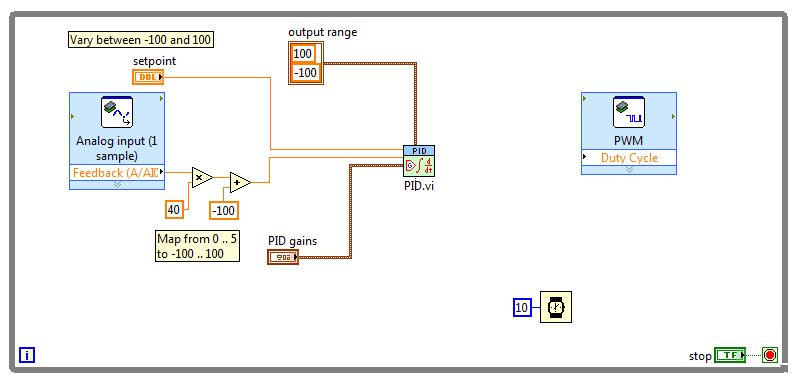##### Figure 13: Scaling the feedback values.

We must also scale the output, which varies between -100 and 100, according to our previously determined duty cycle values: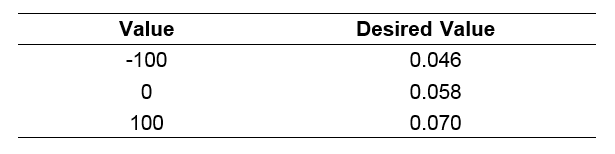##### Table 5: Output (duty cycle) mapping table.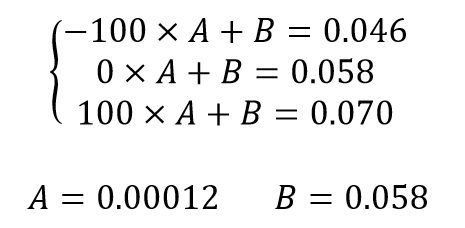##### Figure 14: Solving for the slope (A) and the offset (B) needed for scaling the output values into the range 0.046 to 0.070.

The following figure shows how we incorporate the output scaling into the VI.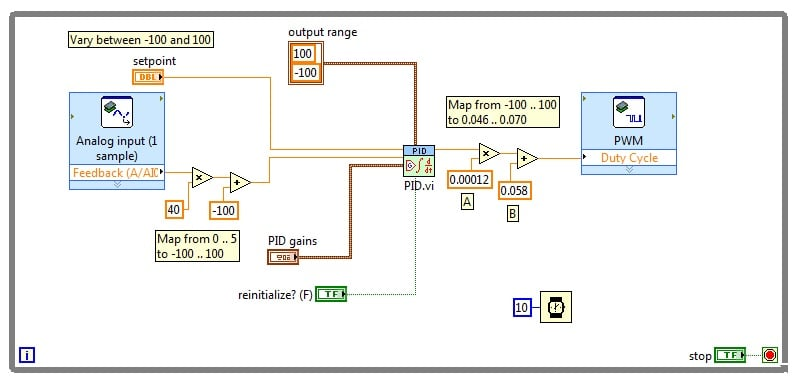#### Summary

If you have followed the tutorial step by step, then you have successfully implemented the PID controller for the servo, bypassing the original servo control circuitry.

If you now switch to the Front Panel of your VI, you can adjust all three PID gains independently and control the servo using the “Setpoint” control. Changing the gains allows you to tune your PID controller and thereby achieve better servo performance.

Also, notice that I have changed the interface to a slider, as shown in Figure 16 below, to have a more natural way of moving the servo.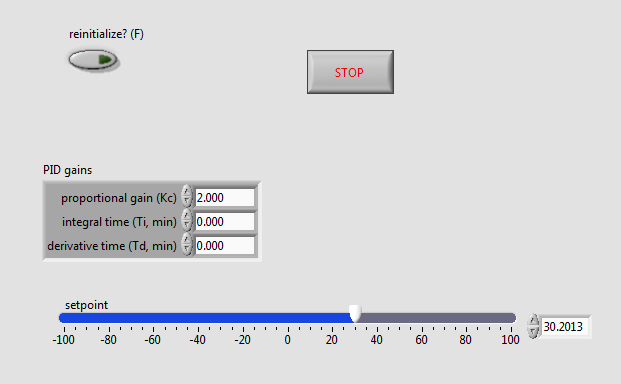##### Figure 16: Front Panel of the final VI for this project.

You can do this, too, by right-clicking on a numeric control and selecting “Replace >> Numeric >> Horizontal Pointer Slide.”

Congratulations on implementing a PID controller using LabVIEW and NI myRIO!•Ali Khan_1516614903 January 22, 2018
•randika2000e October 31, 2020